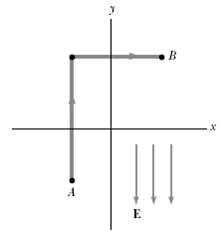# I think I am brain dead today. I can't even solve this simple problem

## Homework Statement

A uniform electric field of magnitude 325 V/m is directed in the negative y direction in Figure P25.9. The coordinates of point A are (-0.200, -0.300) m, and those of point B are (0.400, 0.500) m. Calculate the potential difference VB - VA, using the blue path.## The Attempt at a Solution

$$\Delta V = E \cdot \Delta d$$

$$\Delta d = \sqrt{(0.4 - (-0.2))^2 + (0.5 - (-0.2))^2} = 1$$

$$\Delta V = V_b - V_a = (-325V/m)(1m) = -325V$$

Apparently the actual answer is just the vertical path, that is (-325V/m) * (-0.8m) = 260V

Now my question is, just what am I doing? I did the path from a to b and found the length to be 1.

## Answers and Replies

tiny-tim
Science Advisor
Homework Helper
hi flyingpig!(have a delta: ∆ and a square-root: √)

work done is a dot product of two vectors

∆d is a vector, not a scalar

(you can only treat force and displacement as scalars in a purely 1D situation)

Wait you know what I just overlooked

$$-E \cdot \Delta d = <0,-325> \cdot <0,0.8> = -260V$$

But how did the book get +260V?

tiny-tim
Science Advisor
Homework Helper
you've miscounted the minusesWhat do you mean?

tiny-tim
Science Advisor
Homework Helper
you wrote -E in letters, but then you wrote +E in numbersNo I had <0,-325>

gneill
Mentor
$$dV = - \vec{E} \cdot \vec{ds}$$

tiny-tim
Science Advisor
Homework Helper
No I had <0,-325>

that is +EIs it just me or is PF laggin really hard the last two days? It almost always pops up a dead link for me.

gneill
Mentor
Is it just me or is PF laggin really hard the last two days? It almost always pops up a dead link for me.

Yeah, its very, very slow, and often only gets only partway through loading a page before the browser gives up. It's almost unusable.

It looks for all the world like a denial of service attack is going on on their IP address.